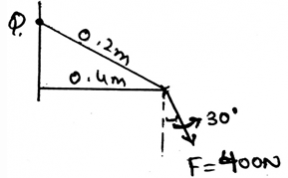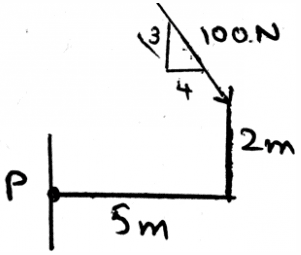Courses

# Test: Frictional Forces On Collar Bearing, Pivot Bearings And Disks

## 15 Questions MCQ Test Engineering Mechanics | Test: Frictional Forces On Collar Bearing, Pivot Bearings And Disks

Description
This mock test of Test: Frictional Forces On Collar Bearing, Pivot Bearings And Disks for Mechanical Engineering helps you for every Mechanical Engineering entrance exam. This contains 15 Multiple Choice Questions for Mechanical Engineering Test: Frictional Forces On Collar Bearing, Pivot Bearings And Disks (mcq) to study with solutions a complete question bank. The solved questions answers in this Test: Frictional Forces On Collar Bearing, Pivot Bearings And Disks quiz give you a good mix of easy questions and tough questions. Mechanical Engineering students definitely take this Test: Frictional Forces On Collar Bearing, Pivot Bearings And Disks exercise for a better result in the exam. You can find other Test: Frictional Forces On Collar Bearing, Pivot Bearings And Disks extra questions, long questions & short questions for Mechanical Engineering on EduRev as well by searching above.
QUESTION: 1

### The pivots are generally used in __________

Solution:

The pivots are being used in the machines. They are used as to support the loadings. This means that the more the pivots the more is the stable the structure. Thus the use of pivots.

QUESTION: 2

### Determine the moment about the point Q by the force shown as 400N.Solution:

As we know that the moment is the cross product of the force and the distance between the point of contact of the force and the point about which moment needs to be calculated. Thus forming the distance vector and then crossing it with the force will give us the answer. Remember force also needs to be in the vector form for doing the cross product.

QUESTION: 3

### The collar bearings are generally used in __________

Solution:

The collar bearings are being used in the machines. They are used as to support the loadings. This means that the more the collar bearings the more is the stable the structure. Thus the use of collar bearings.

QUESTION: 4

The pivots and collar bearings are used as to support the axial load on the rotating shaft.

Solution:

The collar bearings and pivots are being used in the machines. They are used as to support the loadings. This means that the more the collar bearings or pivots more is the stable the structure. Thus used as a supporting system to the shafts.

QUESTION: 5

A phenomena is there in these collar bearings and pivots, which is very helpful in the supporting of the machines, and that is _____________

Solution:

The dry friction is acted upon the surfaces. And they are tangential to each other. As we know the friction is the phenomena that defines that there is a resistance which is present there between the two surfaces. This Coulomb friction is also known as dry friction.

QUESTION: 6

At what conditions does the Coulomb friction occurs between the surfaces in contact of collar bearings or pivots?

Solution:

When there is no lubricating fluid present between the surfaces in contact, the dry friction occurs. Thus as the name suggests, dry friction. When there is no liquid present over there the surfaces are going to be said dry only. Thus the term dry friction.

QUESTION: 7

We first make equilibrium equations of the sections involving the collar bearings or pivots by considering all the three dimensional forces acting on the section chosen and then the free body diagram is made and solved.

Solution:

We first make the free body diagram of collar bearings or pivots and then we make the equilibrium equations to satisfy the given conditions. This helps us to solve the question easily. As this reduces the part of imagination and increases accuracy too.

QUESTION: 8

To know the rotations involved in the disks we use right handed coordinate system, in that system which of the following is followed (consider the mentioned axis to be positive)?

Solution:

As right handed coordinate system means that you are curling your fingers from positive x-axis towards y-axis and the thumb which is projected is pointed to the positive z-axis. Thus visualizing the same and knowing the basic members of axis will not create much problem.

QUESTION: 9

In the calculations involving the collar bearings and the pivot bearings, we use cross product. In that mathematically, for two vectors A and B of any magnitude, the cross product of both, i.e. AxB = given by:

Solution:

The cross product of two vectors give a vector which is perpendicular to both of the vectors. And the mathematic equation for the same is given by: |A||B|sinØ. And the dot product of the same by any of the other two vectors will give the answer zero, as perpendicular. The cross product is same for all the systems of its application.

QUESTION: 10

In the calculations of the bearings we often use vector math. So for those systems which of the following is true?

Solution:

As the mathematic equation for the cross product is having a cosine function in it, in which the angle used in the function is the angle between the vectors. Thus the cross product will be zero if the angle between them is 90. The vector math system remains same for every system in the environment.

QUESTION: 11

The moment is the cross product of which two vectors in the calculations of the pivots and disks?

Solution:

The cross product needs to take in the proper sequence. If not taken then the answer is just the opposite of the true answer. That’s why, the answer is not the Force and Radius vectors, but the Radius and Force vectors. Because the moment has its direction, as many of the cross products have, and thus precaution needs to be taken.

QUESTION: 12

The basic type of motion of a body is translation motion only.

Solution:

The basic types of the motion of the body are translation and rotational. In which translation means the motion is a straight motion while the in the rotational motion the body is moving of the body along the axis. The pivots do circular motion.

QUESTION: 13

Principle of transmissibility is somehow applied to the collar bearings. It states that:

Solution:

Principle of transmissibility states that the force acting on the body is a sliding vector. That is it can be applied at any point of the body. It will give the same effect as if applied at any point other that the specific point. Thus the answer.

QUESTION: 14

Coplanar forces are not easily simplified in the simplification of the force and couple system in the calculations of forces in the pivots and collar bearings.

Solution:

The coplanar forces can be easily simplified in the simplification of the force and couple system. This is not only for the coplanar system of the forces, but for any of the dimension vector. Which means the answer is false.

QUESTION: 15

Determine the moment about the point P.Solution:

As we know that the moment is the cross product of the force and the distance between the point of contact of the force and the point about which moment needs to be calculated. Thus forming the distance vector and then crossing it with the force will give us the answer. Remember force also needs to be in the vector form for doing the cross product.